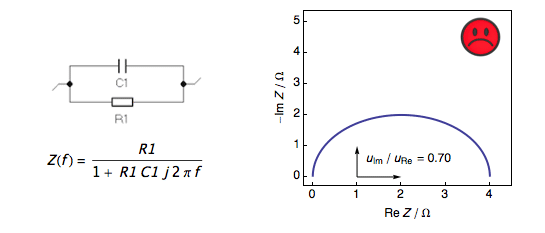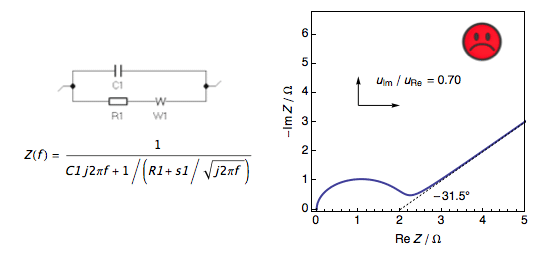Topic 5 min read# Nyquist plots: Why use the orthonormal scale?

Latest updated: August 9, 2023

Introduction

Orthonormal scales should be used for Nyquist impedance plots. The length from 0 to 1 along the imaginary axis should be equal to the length from 0 to 1 along the real axis. Otherwise, semicircle graphs are not semicircles (Fig. 1) and it becomes difficult to measure angles (Fig. 2). We present here two examples: the impedance for a Tafelian redox system (Fig. 1) and the Randles circuit with Warburg impedance (Fig. 2). Orthonormal and non-orthonormal plots are compared: non-orthonormal scales are indicated by a sad face and the orthonormal scale is indicated by a happy face. More details in the corresponding Mathematica Demonstration .Figure 1: Nyquist plot of the impedance of Tafelian redox system showing a
sad face when the scale is not orthonormal and a happy face when the scale
is orthonormal, and the semicircle is a semicircle.Figure 2: Nyquist plot of the impedance of a Randles circuit with Warburg impedance
showing a sad face when the scale is not orthonormal and a happy face when the scale
is orthonormal, and the low-frequency angle is – 45°.

Reference

1. Jean-Paul Diard and Claude Montella “Orthonormal Scales in a Nyquist Diagram” http://demonstrations.wolfram.com/OrthonormalScalesInANyquistDiagram/
Wolfram Demonstrations Project Published: February 26 2019

EIS Nyquist plot orthonormal impedance

## Related products

Work smarter. Not harder.

Tech-tips, theory, latest functionality, new products & more.

No thanks!